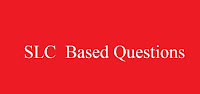### Tuesday, March 1, 2016Slc based question download
Shree Gauri Shankar Higher Secondary School
Third Terminal Examination - 2072
Subject : Computer Science
Class: 10 (Ten)                  Time: 1 hr. 30 mins.         F.M/P.M-50/16

Group A (Fundamental – 22 Marks)
1.      Answer the following questions:                                                                             2 × 5 = 10
a)   Mention the differences between client-server network model and peer-to-peer network model.
b)   What is internet? How is it different than intranet?
c)    Multimedia technology makes classroom presentation more interactive. Explain.
d)    What is meant by defragmentation?
e)    List any four reasons for creating computer viruses.

2.a. Perform the following binary calculations:                                                             2x2=1
i. (101011 x 10) – 11101                   ii. 111001 ¸ 100
b. Convert the following as indicated:                                                                         2x2=2
i. (76)10 = (?)2                                         ii. (AB5)16 = (?)8

3. Match the following.                                                                                      4 × 0.5 = 2
a)      UPS                               Internet protocol
b)      TCP/IP                         Responsible for sending the mails
d)      Apple talk                    Compulsory protocol for computer
Hardware security

4. Replace the following definitions with a technical term.                                         2
a)      A network of computer formed by using cables.
b)     It can be used to authenticate the identity of the sender or the signer of a document.
c)      It is a protocol which helps in bypassing the phone calls using computer.
d)      The process of restoring encrypted data to its original form.

5. Write the full forms of:                                                                                  4 × 0.5 = 2
a. MPEG          b. PSTN                 c. DNS                    d.  RJ – 45

6. Choose the best answer.                                                                                4 × 0.5 = 2
a.   A real time communication service provided by internet is ………….
i.   E-mail               ii. Chat               iii. FTP                    iv. Website

b.  The communication mode where receiver cannot respond immediately.
i.  Half duplex      ii. Full duplex    iii. Simplex            iv. None of the above

c.  Cyber law of Nepal was enacted in Nepal in ………….. B.S.
i. 2062                     ii. 2060               iii. 2061                  iv. 2068

d. A program originally developed to allow users to view document on the WWW.
i. Telnet                   ii. Website          iii. Web browser    iv. web page
Group B (DBMS – 10 Marks)
7. Answer the following questions:                                   2 × 3= 6
a. List any four advantages of DBMS.
b. What is an update query? List any two features of primary key.
c. List any two differences between Form and Report.

8. State whether the given statements are true or false.               4 × 0.5 = 2
a. A field name can have maximum of 64 characters.
b.  The Date/ Time field data type occupies 4 bytes of memory.
c. A field cannot be declared without data type.
d. A row of a table is known as field in MS-Access.

9.      Match the following.                                                                 4 × 0.5 = 2
a.    Hyperlink                           stores unique sequential number
b.    Yes/No                               Stores numbers
c.    Auto number                     stores email address
d.    Number                              stores images
Stores logical data

Group C (Programming – 18 Marks)
10. Answer the following questions:                                                                                3
a. List a difference between Argument and Parameter?
b. Why C language is called middle level language?
c. Write down the function of FILES statements and NAME command.

11. Write the output of the following program.                                             2
DECLARE SUB DISPLAY(X)
CLS
FOR N = 1 TO 10 STEP 3
CALL DISPLAY (N)
NEXT N
END
SUB DISPLAY (X)
IF X MOD 2 =1 THEN PRINT X;
END SUB

12. Rewrite the following program correcting the bugs.                               2
REM to store name and age in a sequential data file STD.DOC
OPEN STD.DOC FOR OUT AS#1
INPUT “Enter name”;N
INPUT “Enter age”;A
WRITE 1,N\$,A
CLOSE #1
END

13. Read the following program and answer the questions:                                                       2              DECLARE SUB TEST (W\$)
CLS
INPUT “ENTER A WORD”;A\$
CALL TEST (A\$)
END
SUB TEST (W\$)
FOR K = 1 TO LEN (W\$)
U\$ = MID\$ (W\$, K, 1) +U\$
NEXT K
PRINT U\$
END SUB
Questions:
a.       List the arguments and parameters used in the above program.
b.       List the library functions used in the above program.

14.a. Write a program that asks a user to input radius of a sphere and then it calls a Sub procedure to display area of the sphere where Area=4πr2 using Sub Procedure.         3
b. WAP to declare a function procedure that checks whether a enter number is prime or composite.                                                                                                            3
c. A data file named “EMP.DAT” contains some records with fields Code, Name, Post and Salary. Write a program to print odd position records of the data file.                        3

****** Best of Luck ******